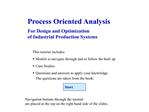# POA Toolbox - PowerPoint PPT PresentationDownload PresentationPOA Toolbox

POA Toolbox
Download Presentation## POA Toolbox

- - - - - - - - - - - - - - - - - - - - - - - - - - - E N D - - - - - - - - - - - - - - - - - - - - - - - - - - -
##### Presentation Transcript

3. Flow Diagram

4. Flow Diagram � Model of a Bar

5. Flow Diagram � Model of a Bar

6. Flow Diagram � Model of a Bar

7. Flow Diagram � Model of a Bar

8. Flow Diagram � Model of a Bar

9. Flow Diagram � Model of a Bar

10. Flow Diagram � Model of a Bar

11. Flow Diagram � Model of a Bar

12. Flow Diagram � Model of a Bar

13. Flow Diagram � Model of a Bar

14. Flow Diagram � Question 1

15. Flow Diagram � Question 1

16. Flow Diagram � Question 1

17. Flow Diagram � Question 2

18. Flow Diagram � Question 2

19. Value Flow Diagram

20. VFD � Case Study of a Weaving Mill

21. VFD � Case Study of a Weaving Mill

22. VFD � Case Study of a Weaving Mill

23. VFD � Case Study of a Weaving Mill

24. VFD � Case Study of a Weaving Mill

25. VFD � Case Study of a Weaving Mill

26. VFD � Investment Analysis

27. VFD � Investment Analysis

28. VFD � Investment Analysis

29. VFD � Investment Analysis

30. VFD � Question 1

31. VFD � Question 1

32. VFD � Question 1

33. VFD � Question 2

34. VFD � Question 2

35. VFD � Question 2

36. VFD � Question 2

37. Resource Flow Diagram

38. RFD � Navigate through model

39. RFD � Navigate through model

40. RFD � Navigate through model

41. RFD � Navigate through model

42. RFD � Navigate through model

43. RFD � Navigate through model

44. RFD � Model of a Gas Station

45. RFD � Model of a Gas Station

46. RFD � Model of a Gas Station

47. RFD � Model of a Gas Station

48. RFD � Model of a Gas Station

49. RFD � Model of a Gas Station

50. RFD � Model of a Gas Station

51. RFD � Model of a Gas Station

52. RFD � Question 1: Mass balance

53. RFD � Question 1: Mass balance

54. RFD � Question 2: Embodied Energy

55. State Chart

56. State Chart � Case Study

57. State Chart � Case Study

58. State Chart � Case Study

59. State Chart � Case Study

60. State Chart � Case Study

61. State Chart � Question 1

62. State Chart � Question 1

63. State Chart � Question 1

64. State Chart � Question 1

65. State Chart � Question 2

66. State Chart � Question 2

67. Simulation Model

68. Simulation Model � Gas Pump

69. Simulation Model � Gas Pump

70. Simulation Model � Gas Pump

71. Simulation Model � Gas Pump

72. Simulation Model � Gas Pump

73. Simulation Model � Question 1

74. Simulation Model � Question 1

75. Simulation Model � Question 1

76. Simulation Model � Question 1

77. Simulation Model � Question 2

78. Simulation Model � Question 2

79. Simulation Model � Question 2

80. Simulation Model � Question 2

81. Simulation Model � Question 2

82. Real-Time Control

83. Real-Time Control � Reengineering

84. Real-Time Control � Reengineering

85. Real-Time Control � Reengineering

86. Real-Time Control � Reengineering

87. Real-Time Control � Question 1

88. Real-Time Control � Question 1

89. Real-Time Control - Question 2

90. Real-Time Control - Question 2

91. Real-Time Control - Question 2